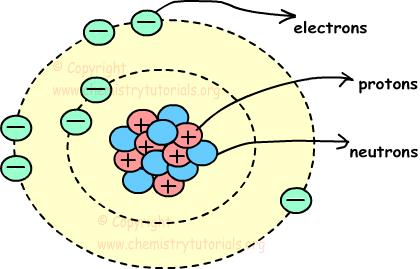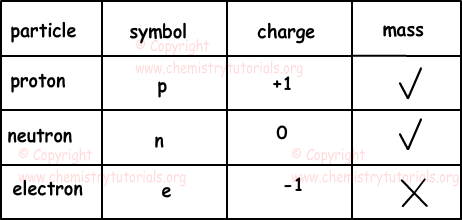## Atomic Structure with Examples

Atomic Structure with Examples

Atom is the smallest particle of matter. It consist of three particles, called proton, electron and neutron. Protons and neutrons are placed at the center of the atom and electrons are placed around the center. Picture given below shows, structure of atom and locations of proton neutron and electron in atom.Center of the atom, including protons and neutrons, is called also nucleus and circles around nucleus are called orbits.

Protons: Protons are positively charged particles of atoms. They are located in nucleus, and have mass. We show protons with letter "p".

Electrons: Electrons are negatively charged moving particles of atoms. They are located around the nucleus on orbits, and have no mass. We show electrons with letter "e".

Neutrons: Neutrons are charge-less particles of atoms. They are located in nucleus, and have mass. We show neutrons with letter "n".Atomic Number:

Atomic number shows the number of protons in each atom. It is specific for each atom and shown with letter "Z". In neutral atom number of protons is equal to number of electrons. Thus;

Z=p=e

Atomic number is written as;

atomic numberX

Mass Number:

Mass of the neutron is approximately equal to the mass of proton. We neglect mass of electron since it is too small. Thus, mass of atom is equal to the sum of mass of protons and neutrons. We show mass number with letter "M". Mass number is not specific for each atom. Different atoms can have same mass number.

M=n+p

We show M on atom as;

ZMX

Isotopes:

Isotopes have same atomic number but different mass number. This means that atoms have same number of protons but different number of neutrons. Since the number of protons are equal, chemical properties of the atoms are same, and since the neutrons numbers are different physical properties of atoms are different. Isotopes of Hydrogen are given below;

11H,

12H ,

13H

Isotones:

Isotone atoms have same  number of neutrons and different numbers of protons.

Sodium and Magnesium are isotone atoms, their numbers of neutrons are equal.

1123Na and 1224Mg

Isobars:

Isobar atoms have different atomic number and different neutron number but same mass number.

1124Na and 1224Mg

Since the protons number and neutrons number of atoms are different, their physical and chemical properties are also different.

Example: Which one of the following statements are same for isotope atoms

I. Number of protons

II. Number of neutrons

III. Number of electrons

IV. Atomic Number

V. Chemical properties

VI. Physical Properties

Isotopes have same atomic number bur different mass number. This means that, isotopes atoms have equal number of protons and different number of neutrons. If the atom is neutral then number of protons in the atom is equal yo number of electrons. Since the number of protons of atoms are equal and number of neutrons are different; chemical properties of these atoms are same but physical properties of them are different. Thus;

I. III. IV. and V is true for isotopes atoms.

Example: Find the number of protons of atom having mass number 65 and neutron number 35.

Mass number is equal to sum of protons and neutrons.

M=p+n

65=35+p

p=30

Example: Which ones of the following statements are true for 1224X and 1124Y atoms.

I. Number of protons X>Y

II. neutron number of Y is larger than neutron number of X

III. Their mass numbers are equal

1224X :

X has 12 protons and

24-12=12 neutrons

Mass number=24

124Y:

Y has 11 protons and

24-11=13 neutrons

Mass number=24

Thus, I. II. and III. are true.

Example:

I. 2656Fe+2, 2656Fe+3

II. 2656Fe, 2756Co

III. H2O, D2O

Which one of the couples given above has same chemical properties. (11H, 12D)

To have same chemical properties, they must have equal numbers of protons and electrons.

I. Both of them has same numbers of proton 26, but their electron numbers are different. First one has 26-2=24 electrons and second one has 26-3=23 electrons. Thus they have different chemical properties.

II. Since both of them have different numbers of protons and electrons, they have different chemical properties.

III. In this couple, they have equal number of protons and electrons they have same chemical properties.

Atomic Structure Exams and  Problem Solutions

Related